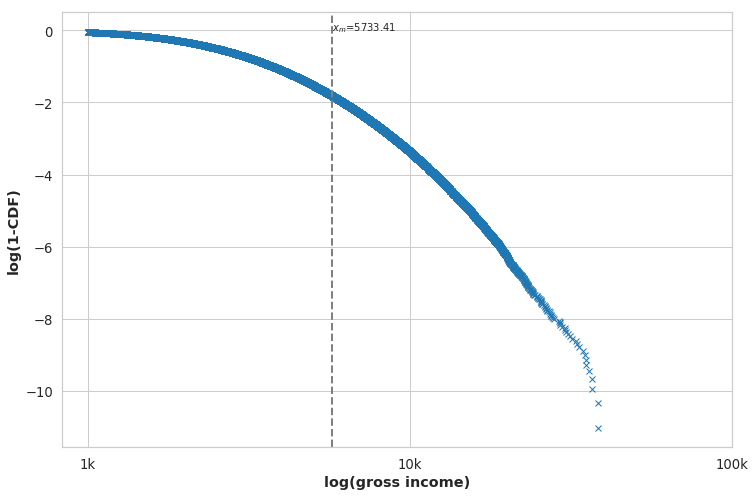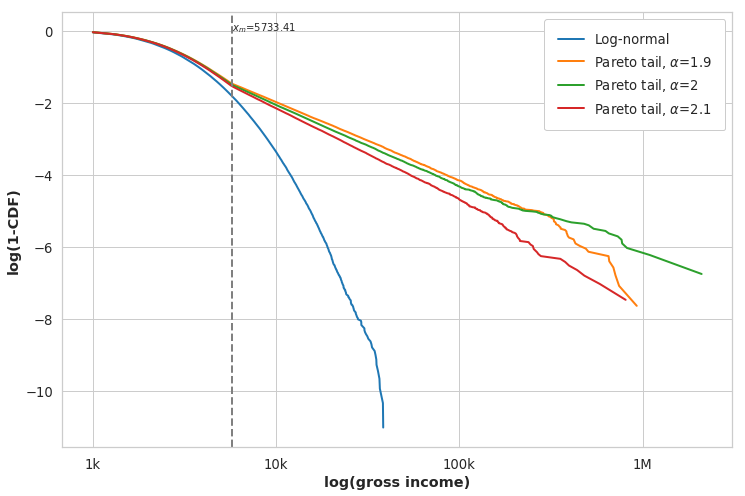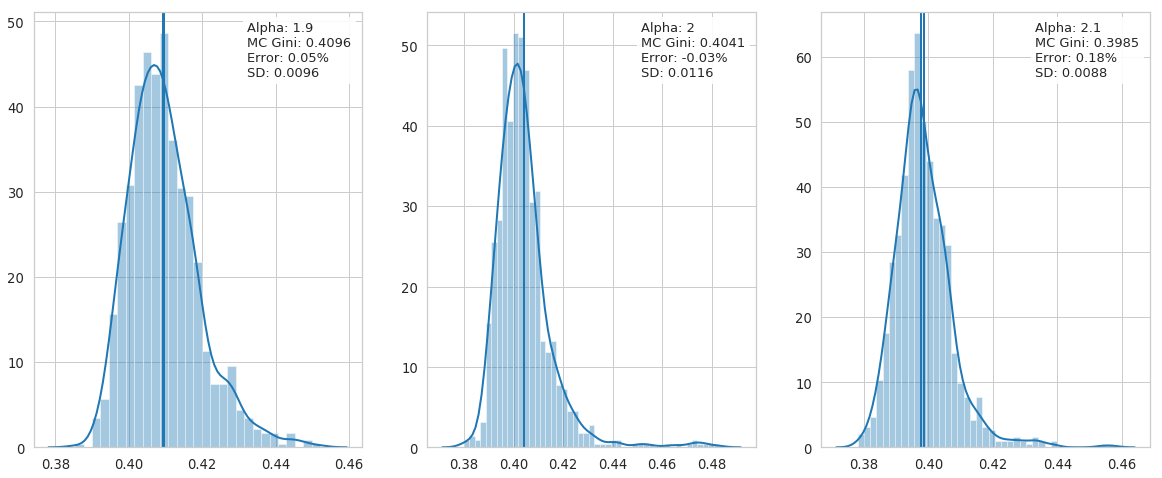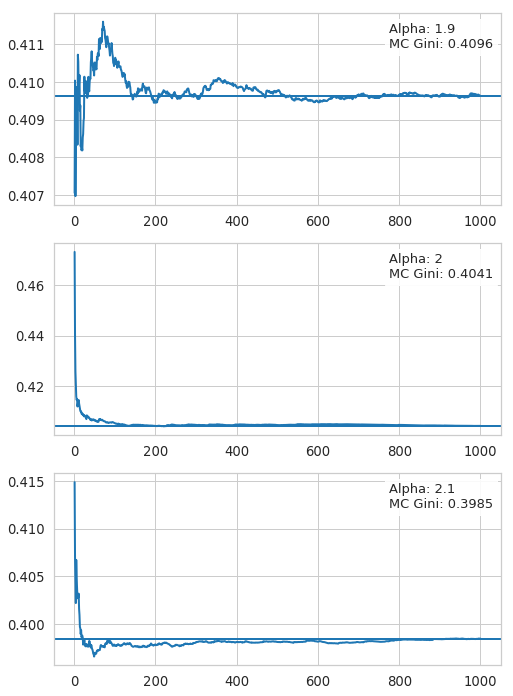# Motivation¶

When I reach back when I got interested in Economics I think it started around six years ago. I remember that my first book that got me into this topic was R. Rajan's Fault Lines: How Hidden Fractures Still Threaten the World Economy. I've read it during my stay as an intern in the European Parliament. I was moved by the argument that growing inequality in the US created political pressure for easy credit to fabricate the illusion of wealth, where there was none. This in consequence led to the housing bubble ending in the Financial Crash of 2007 and the depression that came afterwards.

From that point I got interested in inequality. I've started to read a lot of books and scientific papers on inequality and income distribution. I was hooked by an article Dragulescu, A. & Yakovenko, V., Statistical mechanics of money, Eur. Phys. J. B (2000) 17: 723., where the authors simulated income distributions from simple physics law that I knew well from studying chemistry. I wanted to know more and I also run my own simulations. I enjoyed it so much that I enrolled and got into PhD in Economics in Poznań Economic University. I was surprised that not much information on this topic existed in Poland by that time. By total accident I found one report by my current employer, CenEA that actually had something on income distribution in Poland. It was interesting enough that I wanted to work with them. I still do.

Recently I got to work on income distribution in Poland based on a paper by P. Bukowski & F. Novokmet, where the authors interpolate Poland's top income percentiles time series since the beginning of the XIX century.

This work has brought some questions including the behavior of Gini coefficients under sampling a log-normal distribution with a Pareto tail. I'm curious what happens with the Gini coefficient during sampling from a known population.

# Setup¶

I will simulate a random variable $X_l$ from a log-normal distribution. Next I will change the top 5% of results with random variables $X_p$ coming from a Pareto distribution with a PDF: $$p(x) = \frac{\alpha x_m^\alpha}{x^{\alpha+1}}\ for\ x \ge x_m$$ and CDF: $$F(X) = 1 - \left(\frac{x_m}{x}\right)^\alpha for\ x \ge x_m$$ With $x_m = p_{95}$ being the 95th percentile.

I will set $\alpha = \{1.9, 2, 2.1\}$. Case where $\alpha$ is equal or less than two is interesting, because in this setup the Pareto distribution has infinite variance. I want to check how it will influence Gini coefficient with sampling.

I will do all the calculations in Python.

In :
import warnings
warnings.simplefilter(action='ignore', category=FutureWarning)
import matplotlib.pyplot as plt
%matplotlib inline

import numpy as np
import pandas as pd
import seaborn as sns
from scipy.special import erf
from scipy import stats

# Make graphs look good
sns.set_style("whitegrid")

plt.rcParams['figure.figsize'] = (12,8)
plt.rcParams['axes.labelweight'] = 'bold'

sns.set_context("notebook", font_scale=1.2, rc={"lines.linewidth": 2.0,
'legend.frameon':True,
'figure.figsize': (12,8)})

In :
# Set constant seed for reproducibility
np.random.seed(123456)

In :
pop_size = 1000000

mu = 7.566731
sigma = .66069957

data = pd.DataFrame({'population' : np.exp(np.random.normal(mu, sigma, pop_size)) })

pctiles = np.percentile(data.population, [50, 90, 95, 99] )
print(pctiles)

[1933.65602304 4506.67661963 5733.40668502 9020.96857301]

In :
def gen_plot_cdf(d):
d_sort = np.sort(d)
cdf = np.cumsum(d_sort) / np.sum(d_sort)
lcdf = np.log(np.ones(cdf[:-1].shape)-cdf[:-1])
ld = np.log(d_sort[:-1])
return ld, lcdf

In :
ld, lcdf = gen_plot_cdf(data.population)

plt.figure(figsize=(12,8))
plt.plot(ld[ld>np.log(1e3)], lcdf[ld>np.log(1e3)], 'x')
plt.xticks([np.log(x) for x in [1e3, 1e4, 1e5]], ["1k", "10k", "100k"])
plt.axvline(x=np.log(pctiles), c="gray", ls="--")
plt.xlabel("log(gross income)")
plt.ylabel("log(1-CDF)")
plt.text(np.log(pctiles), 0,  '$x_m$={:.2f}'.format(pctiles))

Out:
Text(8.65407,0,'$x_m$=5733.41')I substitute here values above the $p_{95}$ threshold with values coming from the Pareto distribution with three parameters. I do this in a loop where first I copy the log-normal distribution, then I substitute only values greater than the threshold. Numpy has a numpy.random.pareto function that returns values from 0 to $+\inf$. In the end I need to add one and multiply by my scale parameter $x_m = p_{95}$.

In :
alphas = [1.9, 2, 2.1]

high_incs = data['population'] >= pctiles

for alpha in alphas:
iname = 'inc' + str(alpha)
data[iname] =  data['population']
data[iname][high_incs] = (1+np.random.pareto(alpha, high_incs.shape)) * pctiles


Plotting old and new distributions gives us the next figure. We can see that the Pareto distribution in this graph is a straight line. When we take the $\log$ of both sides we end up with a linear equation.

$$F(X) = 1 - \left(\frac{x_m}{x}\right)^\alpha for\ x \ge x_m$$$$1 - F(X) = \left(\frac{x_m}{x}\right)^\alpha$$$$\log\left( 1 - F(X) \right)= \alpha \log \left( x_m \right) - \alpha \log \left( x \right)$$
In :
plt.figure(figsize=(12,8))
plt.plot(ld[ld>np.log(1e3)], lcdf[ld>np.log(1e3)], label="Log-normal")
for alpha in alphas:
inc = 'inc' + str(alpha)
p_ld, p_lcdf = gen_plot_cdf(data[inc])
plt.plot(p_ld[p_ld>np.log(1e3)], p_lcdf[p_ld>np.log(1e3)], label="Pareto tail, $\\alpha$=%s"%(alpha))

plt.xticks([np.log(x) for x in [1e3, 1e4, 1e5, 1e6]], ["1k", "10k", "100k", "1M"])
plt.axvline(x=np.log(pctiles), c="gray", ls="--")
plt.text(np.log(pctiles), 0,  '$x_m$={:.2f}'.format(pctiles))
plt.xlabel("log(gross income)")
plt.ylabel("log(1-CDF)")

Out:
<matplotlib.legend.Legend at 0x7f1c2fbcdf28>## Calculating Gini coefficient¶

Unfortunately I could not find inequality measure functions for Numpy and Scipy libraries. Python was designed as a general programming language and by default lacks the statistical bells ans whistles available in Stata or R. But given its flexibility I can easily define my own function. I will be using the same implementation that I use in Stata, namely sgini developed by P. Van Kerm.

It uses a simple formula for calculating Gini using covariance.

$$\operatorname{Gini}(X) = -2 \operatorname{Cov}\left( \frac{X}{\overline{X}}, 1-F\left( X \right) \right)$$

With $\overline{X}$ equal to the mean of our $X$ and $F(X)$ being the CDF.

In :
def gini(inc):
sort_inc = np.sort(inc)
sum_inc = np.sum(sort_inc)
cdf = np.cumsum(sort_inc) / sum_inc
mu = sum_inc / inc.shape
return -2 * np.cov(sort_inc / mu, 1-cdf)[0, 1]



Also for the log-normal distribution there exists a simple way to calculate the Gini coefficient analytically, $G = \operatorname{erf} \left(\frac{\sigma}{2}\right)$. Where $\sigma$ is the same value we used to construct the log-normal distribution. The analytical Gini for our log-normal distribution is close to the Gini calculated on our random variable. We can also observe that the Gini is lower with higher $\alpha$ for the Pareto tail.

In :
g_lognormal_pop = gini(data['population'])
print("Gini lognormal: ", g_lognormal_pop)
print("Gini lognormal, analytical: ", erf(sigma/2))
print("Error: ", (erf(sigma/2) - g_lognormal_pop) / erf(sigma/2))

pop_ginis = []
for alpha in alphas:
inc = 'inc' + str(alpha)
g_pop = gini(data[inc])
pop_ginis.append(g_pop)
print("Gini lognormal + pareto tail, a={}: {:.4f}".format(alpha, g_pop))

Gini lognormal:  0.3598313356200834
Gini lognormal, analytical:  0.3596325801243393
Error:  -0.0005526626527423896
Gini lognormal + pareto tail, a=1.9: 0.4094
Gini lognormal + pareto tail, a=2: 0.4042
Gini lognormal + pareto tail, a=2.1: 0.3978


## Bootstrapping¶

Next I want to sample my population of incomes by taking 1% of all observations and then calculating Gini coefficients on each sub-sample. I take 1000 random draws for each of the $\alpha$ parameters. Below figures show histograms of such values. The mean value of Gini for each parameter is close to the value for the population. That is nice!

In :
gini_mc = {}
for alpha in alphas:
inc = 'inc' + str(alpha)
gini_mc[inc] = [gini(data[inc].sample(frac=0.01)) for x in range(0, 1000)]

In :
fig, ax = plt.subplots(1, 3, figsize=(20,8))

for p in range(0, 3):
inc = 'inc' + str(alphas[p])
sns.distplot(gini_mc[inc], ax=ax[p]) # , fit=stats.beta)
g_mean = np.mean(gini_mc[inc])
ax[p].axvline(x=g_mean)
ax[p].axvline(x=pop_ginis[p])
#plt.text(np.log(pctiles), 0,  '$x_m$={:.2f}'.format(pctiles))

g_text = "Alpha: {}\nMC Gini: {:.4f}".format(alphas[p], g_mean)
g_text += "\nError: {:.2%}\nSD: {:.4f}".format((g_mean-pop_ginis[p])/pop_ginis[p], np.std(gini_mc[inc]))

ax[p].text(0.65, .98 ,g_text, fontsize=13,
horizontalalignment='left',
verticalalignment='top',
transform = ax[p].transAxes,
bbox=dict(facecolor='white', alpha=0.9))I also want to look how the mean value of Gini converges with each additional observations. I just want to make sure that there are no outlier observations that drive the whole mean away. This behavior can somehow be expected by random variables coming from fat-tailed distributions. The figures below show that this is not the case.

In :
fig, ax = plt.subplots(3,1, figsize=(8, 12))

for p in range(0, 3):
inc = 'inc' + str(alphas[p])

cs = np.cumsum(gini_mc[inc]) / np.array(range(1, len(gini_mc[inc])+1))

ax[p].plot(range(1, len(gini_mc[inc])+1), cs)
ax[p].axhline(y=np.mean(gini_mc[inc]))

g_text = "Alpha: {}\nMC Gini: {:.4f}".format(alphas[p], np.mean(gini_mc[inc]))

ax[p].text(0.75, .95 ,g_text, fontsize=13,
horizontalalignment='left',
verticalalignment='top',
transform = ax[p].transAxes,
bbox=dict(facecolor='white', alpha=0.9))# Conclusions¶

Of course this short exercises cannot serve as an official proof that we can bootstrap Gini coefficient for our population based on sub-samples. It is not a mathematical proof. It is just a simple illustration, that this method can work. It does not say anything about the general case.

It is reassuring to know that I can try to sample distributions in such a way. Maybe sometime later I will try to approach it in a more analytical, formal way. But I'll leave it to some other time.